# Plotting track and trajectory of tropical cyclones on a topographic map in Python (codes included)

Short demonstration of how to plot the track or trajectory of a hurricane on a map. Codes are included.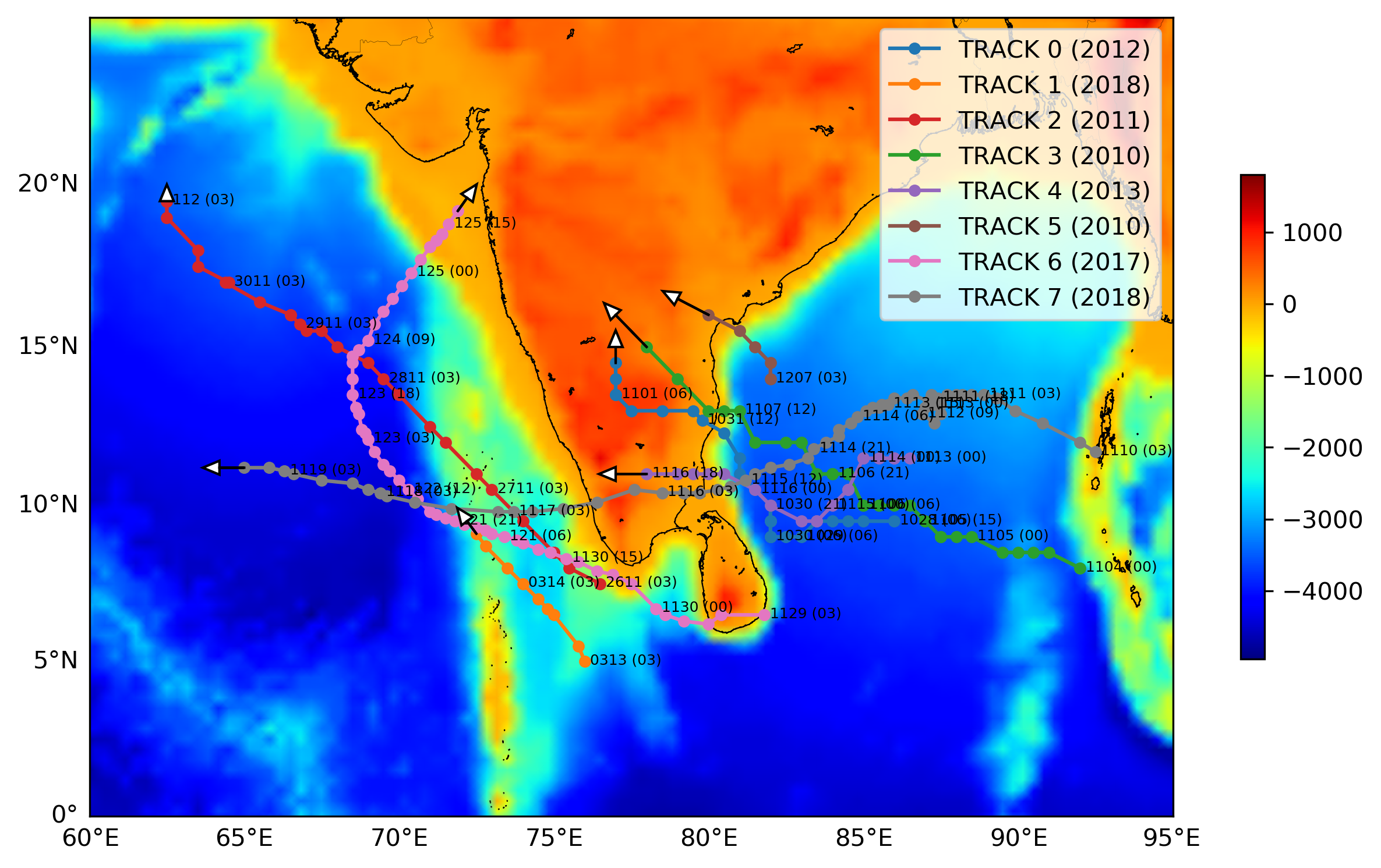## Introduction

Plotting track or trajectory of the hurriance is essential part of analyzing and understanding the hurricane. For details see references.

## Visualization

### Importing Libraries

The first thing I like to do is to import all the necessary libraries for the task. This keeps the code organized.

``````import matplotlib.pyplot as plt
import numpy as np
from mpl_toolkits.basemap import Basemap
import pandas as pd
import glob
from plotting_topo import plot_topo
``````
• `matplotlib.pyplot` is imported for the plotting purpose,
• `numpy` is for scientific computation and operations,
• `Basemap` is the matplotlib library for plotting 2D data on map,
• `glob` is for reading the files present in the directory,
• `plot_topo` is the function that reads the topo data for plotting on the map.

The `topo` dir is location in the current directory.

## Similar posts

### Define map boundary parameters

``````lonmin, lonmax = 60, 95
latmin, latmax = 0, 25
``````

### Set up basemap with topography

For more details of plotting topography on a map, see this post for details: https://www.earthinversion.com/station_map_python/

• instantiate the basemap instance within the defined map boundary. Here, we chose the mercator projection. For other projections, check here
``````fig = plt.figure(figsize=(10,6))
m = Basemap(projection='merc', resolution="f", llcrnrlon=lonmin, llcrnrlat=latmin, urcrnrlon=lonmax, urcrnrlat=latmax)
``````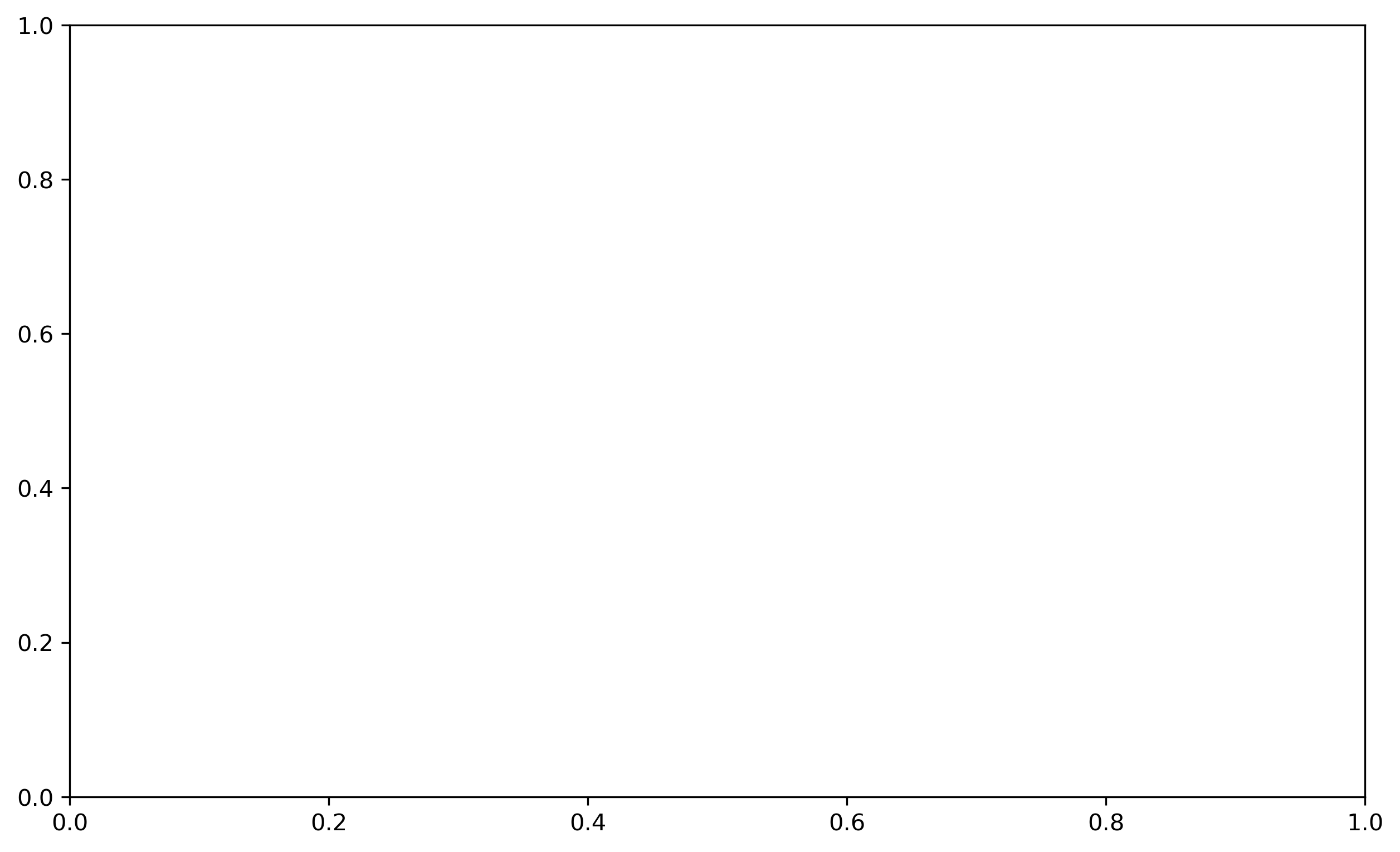• plot the topography on the basemap and draw colorbar
``````cs = plot_topo(m,cmap=plt.cm.jet,zorder=2,lonextent=(lonmin, lonmax),latextent=(latmin, latmax))

fig.colorbar(cs, ax=axx, shrink=0.6)
``````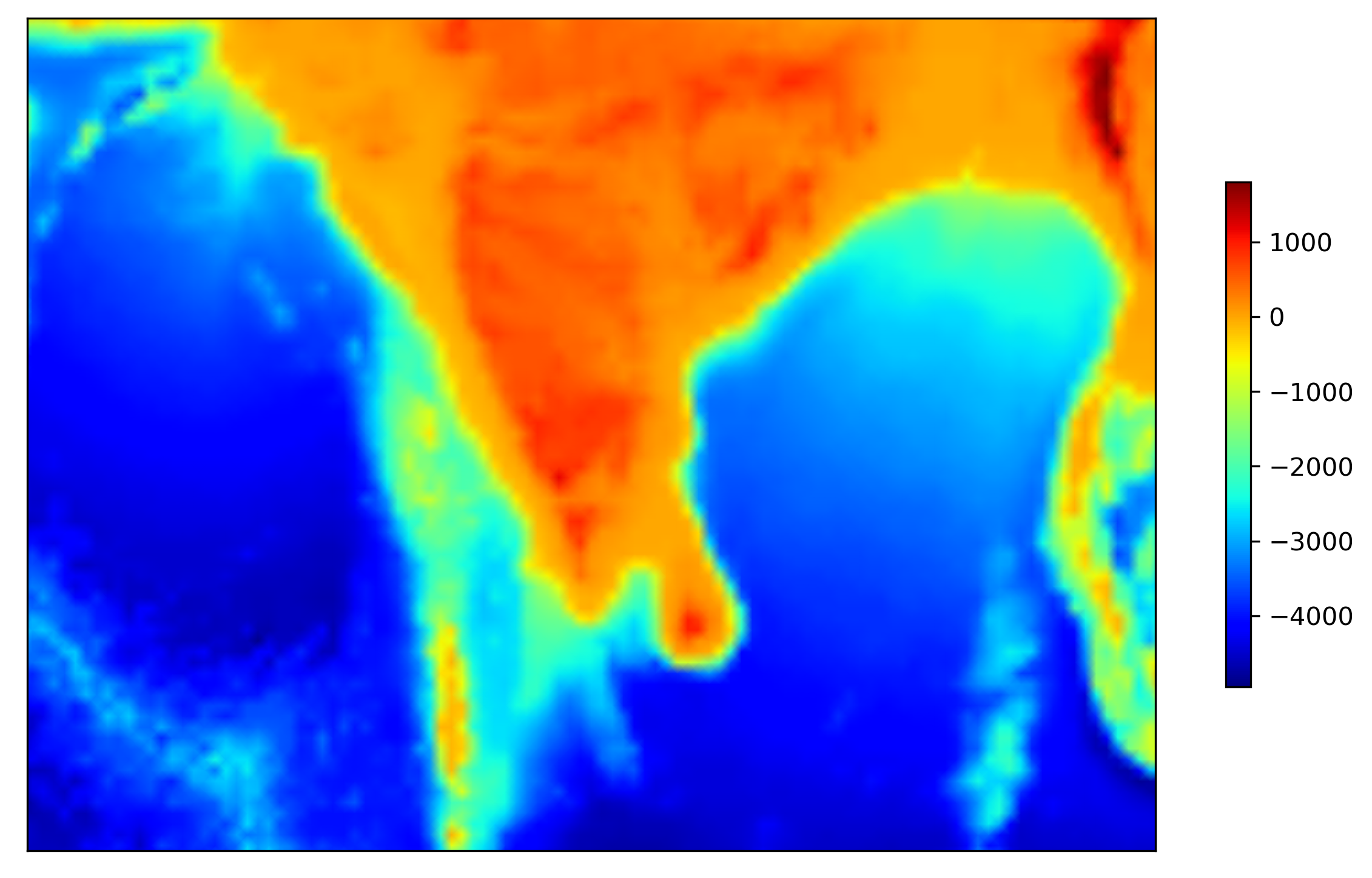• draw latitudinal and longitudinal grid lines with the step of 5 degrees. We only show the labels on the left and bottom of the map.
``````m.drawcoastlines(color='k',linewidth=0.5,zorder=3)
m.drawcountries(color='k',linewidth=0.1,zorder=3)

parallelmin = int(latmin)
parallelmax = int(latmax)+1
m.drawparallels(np.arange(parallelmin, parallelmax,5,dtype='int16').tolist(),labels=[1,0,0,0],linewidth=0,fontsize=10, zorder=3)

meridianmin = int(lonmin)
meridianmax = int(lonmax)+1
m.drawmeridians(np.arange(meridianmin, meridianmax,5,dtype='int16').tolist(),labels=[0,0,0,1],linewidth=0,fontsize=10, zorder=3)
``````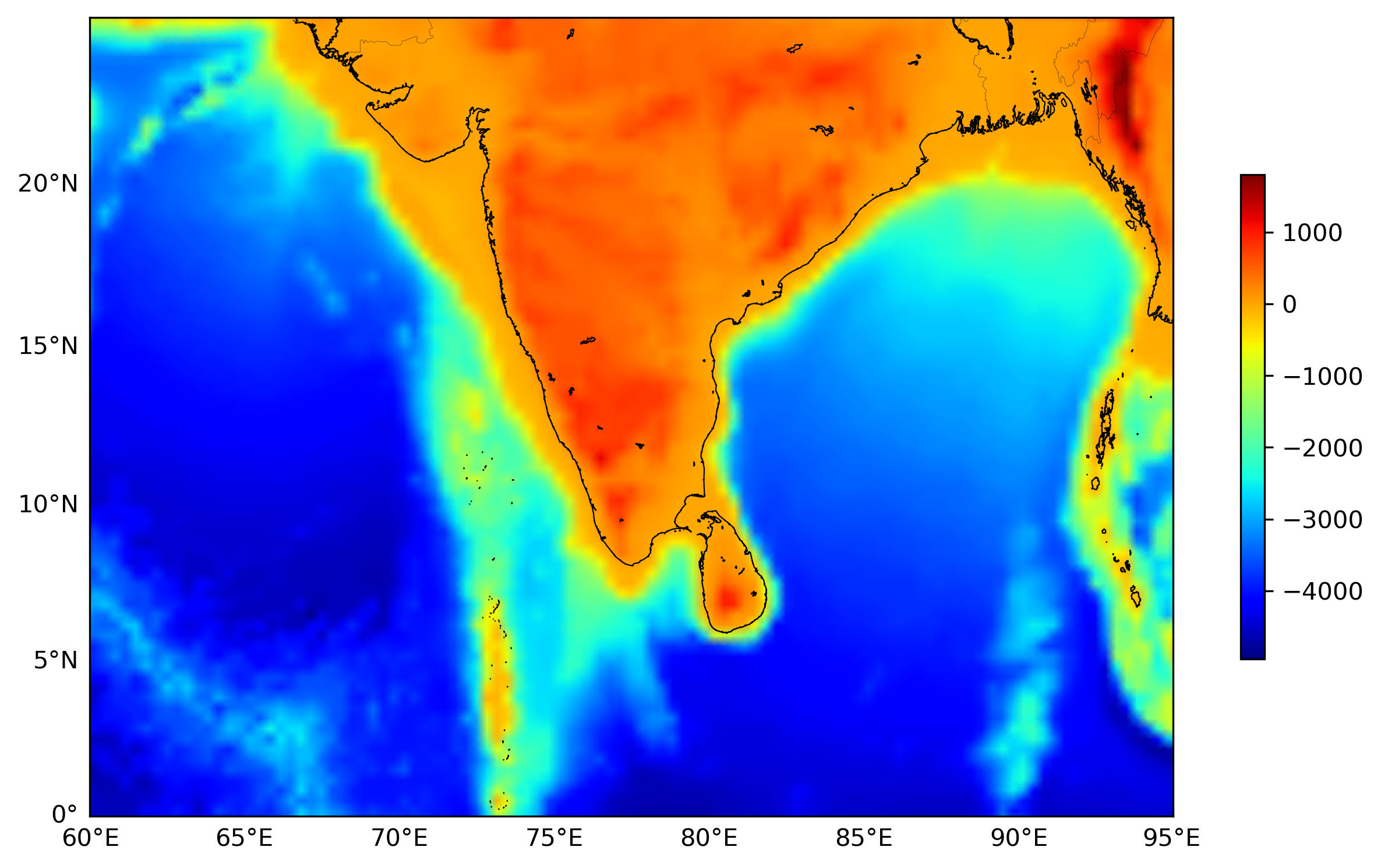### Plot track on the basemap

• define the list of colors to be used for different hurriances
• read the file names stored in the directory `01_TRACKS`, and with the suffix `.txt`
• read the data file as a pandas data frame.
• extract the `year` info from the filename
• we “capitalize” the track name
• get longitude and latitude as numpy array
• convert lat and lon to map projection scale
• plot the track with `-o` and label the name of each track
``````colors = ['C0','C1','C3','C2','C4','C5','C6','C7'] #default colors from Python (can be automated if the order is not important)
datafiles = glob.glob("01_TRACKS/*.txt") #to read individual data files containing the coordinates of the track for each typhoon
for jj in range(8):
dff = pd.read_csv(datafiles[jj],sep='\s+', dtype={'time': object}) #read data file and time as string
year = datafiles[jj].split("/").split("_").split("-") #extract year information from the filename
track_name = datafiles[jj].split("/").split("(").split(")") #extract track information from the filename
track_name = track_name.capitalize()

## extract lat and lon info from pandas data frame and convert to map scale
lons = dff['lon'].values
lats = dff['lat'].values
x, y = m(lons, lats)

## plot the track
m.plot(x, y,'o-',color=colors[jj],ms=4, zorder=4,label=f"TRACK {jj} ({year})")
``````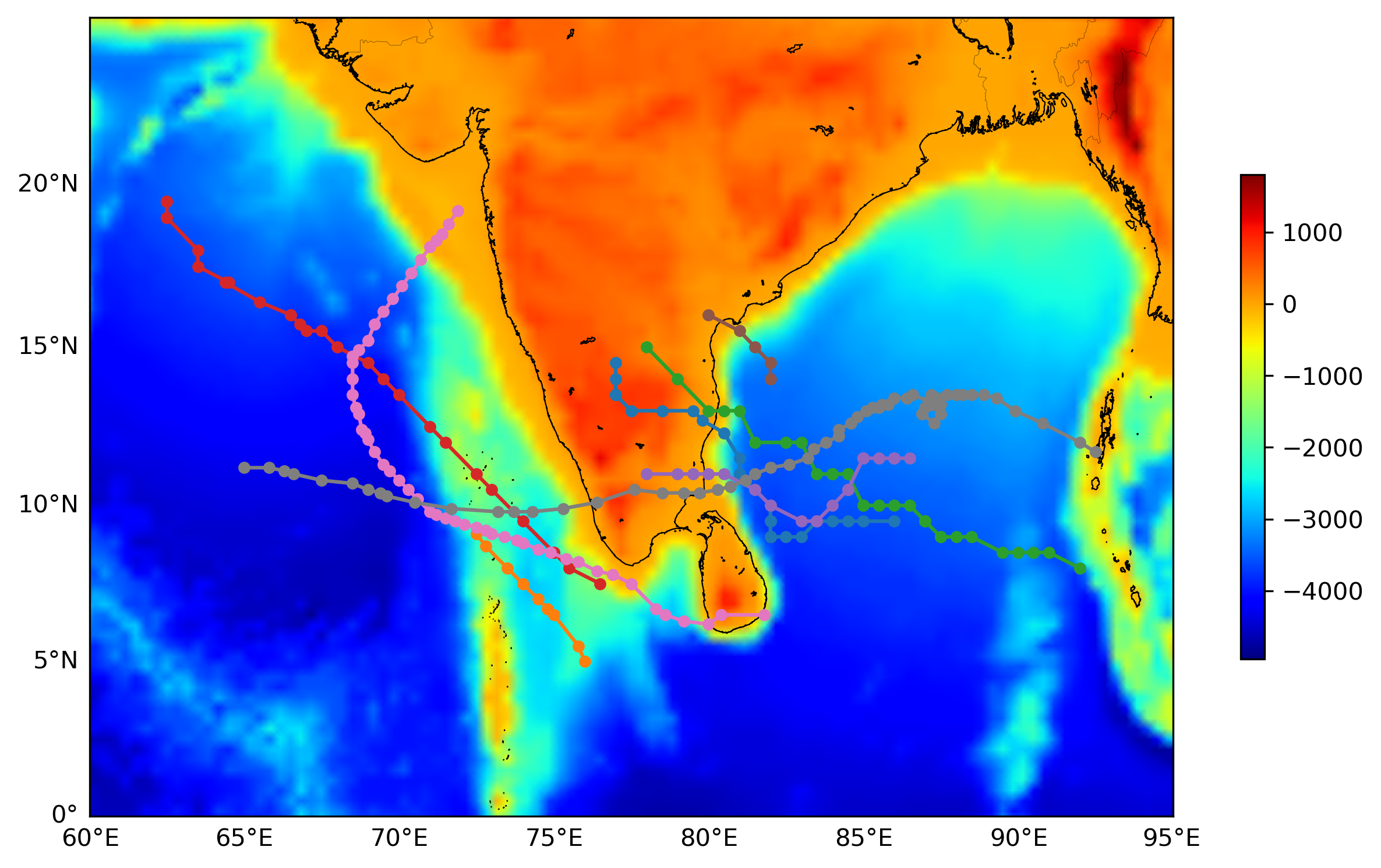• we extract the time at each data point from the data file for plotting on the map. This gives an idea of the direction of the hurricane.
• the extracted values are the date and month and hour.
• the track time is plotted for every 3rd data point with some shift in x and y direction. The shift is given in terms of the map scale.
``````for jj in range(8):
######CONTINUE FROM ABOVE####
#############################
## extract the time info and label the track with the time for every 3 data points
typh_time=[]
for i in range(dff.shape):
date=dff.loc[i,'date']
try:
dd = date.split(".")
month = date.split(".")
except:
dd = date.split("/")
month = date.split("/")

time=dff.loc[i,'time']

track_times="{}{} ({})".format(month, dd, time[:2])
typh_time.append(track_times)
typh_time=np.array(typh_time)

for i in np.arange(0,len(typh_time),5):
plt.text(x[i]+20000,y[i]-10000,typh_time[i],fontsize=6,zorder=4)
``````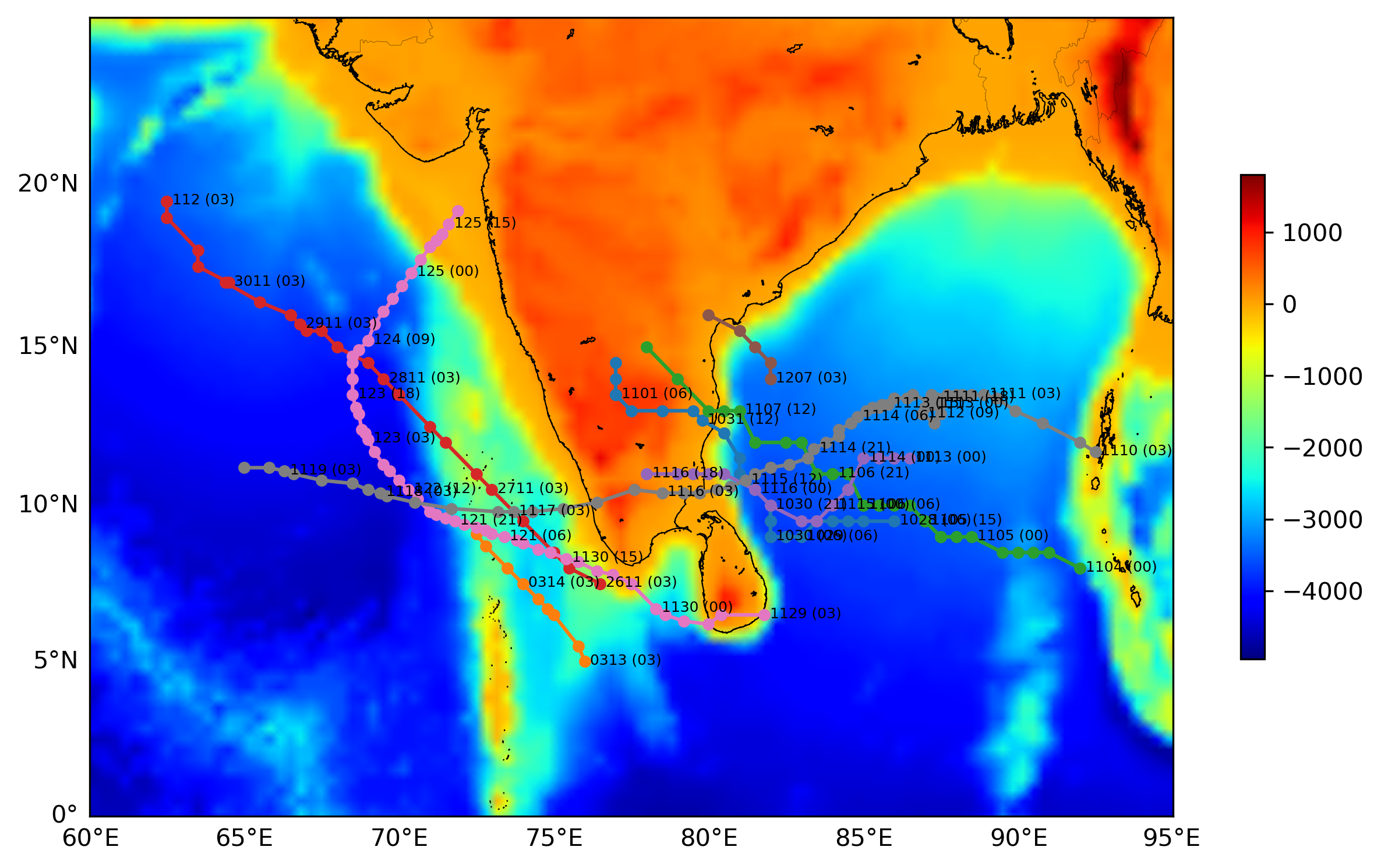• we can put a triangle at the end of the track to indicate the direction of the hurricane
``````for jj in range(8):
######CONTINUE FROM ABOVE####
#############################
## plot the arrow based on the last two data points to indicate the trajectory of the track
``````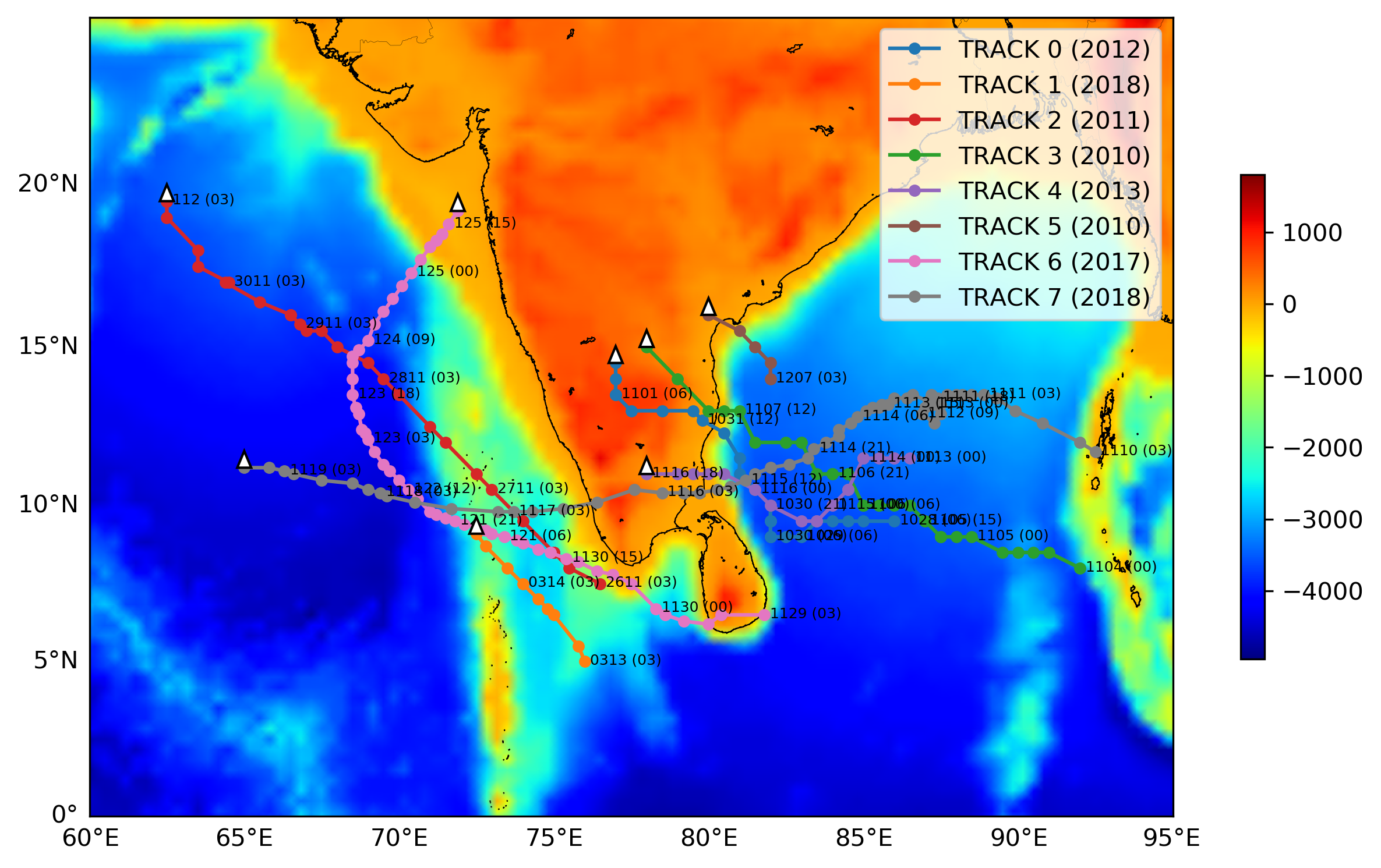• Instead of just triangle to indicate the direction, we plot the rough trajectory of the hurricane based on the last two data points.
``````for jj in range(8):
######CONTINUE FROM ABOVE####
#############################
## plot the arrow based on the last two data points to indicate the trajectory of the track

plt.legend(loc=1)

## save the map as png
plt.savefig('map.png',bbox_inches='tight',dpi=300)
``````1. Janapati. J., B. K. Seela, P.-L. Lin, P. K. Wang, and U. Kumar, 2019: An assessment of tropical cyclones rainfall erosivity for Taiwan. Sci. Rep., 9, 15862. https://doi.org/10.1038/s41598-019-52028-5
2. Janapati. J., B. K. Seela, P.-L. Lin, P. K. Wang, C.-H. Tseng, K. K. Reddy, H. Hashiguchi, L. Feng, S. K. Das, and C. K. Unnikrishnan (2020), Raindrop size distribution characteristics of Indian and Pacific ocean tropical cyclones observed at India and Taiwan sites. Journal of the Meteorological Society of Japan. 98(2), 299−317. https://doi.org/10.2151/jmsj.2020-015
3. Janapati. J., Balaji Kumar seela, M. Venkatrami Reddy, K. Krishna Reddy, Pay-Liam Lin, T. Narayana Rao and Chian-Yi Liu, (2017), A study on raindrop size distribution variability in before and after landfall precipitations of tropical cyclones observed over southern India, J. Atmos. and Sol.-Terr. Phys., Vol. 159, 23–40. https://doi.org/10.1016/j.jastp.2017.04.011
4. Fovell, R. G., and Su, H. (2007), Impact of cloud microphysics on hurricane track forecasts, Geophys. Res. Lett., 34, L24810, doi:10.1029/2007GL031723.
5. Huang, Y., C. Wu, and Y. Wang, 2011: The Influence of Island Topography on Typhoon Track Deflection. Mon. Wea. Rev., 139, 1708–1727, https://doi.org/10.1175/2011MWR3560.1.